JEE  >  Test: Single Correct MCQs: Some Basic Concepts of Chemistry | JEE Advanced

# Test: Single Correct MCQs: Some Basic Concepts of Chemistry | JEE Advanced

Test Description

## 28 Questions MCQ Test Chemistry for JEE | Test: Single Correct MCQs: Some Basic Concepts of Chemistry | JEE Advanced

Test: Single Correct MCQs: Some Basic Concepts of Chemistry | JEE Advanced for JEE 2023 is part of Chemistry for JEE preparation. The Test: Single Correct MCQs: Some Basic Concepts of Chemistry | JEE Advanced questions and answers have been prepared according to the JEE exam syllabus.The Test: Single Correct MCQs: Some Basic Concepts of Chemistry | JEE Advanced MCQs are made for JEE 2023 Exam. Find important definitions, questions, notes, meanings, examples, exercises, MCQs and online tests for Test: Single Correct MCQs: Some Basic Concepts of Chemistry | JEE Advanced below.
Solutions of Test: Single Correct MCQs: Some Basic Concepts of Chemistry | JEE Advanced questions in English are available as part of our Chemistry for JEE for JEE & Test: Single Correct MCQs: Some Basic Concepts of Chemistry | JEE Advanced solutions in Hindi for Chemistry for JEE course. Download more important topics, notes, lectures and mock test series for JEE Exam by signing up for free. Attempt Test: Single Correct MCQs: Some Basic Concepts of Chemistry | JEE Advanced | 28 questions in 50 minutes | Mock test for JEE preparation | Free important questions MCQ to study Chemistry for JEE for JEE Exam | Download free PDF with solutions
 1 Crore+ students have signed up on EduRev. Have you?
Test: Single Correct MCQs: Some Basic Concepts of Chemistry | JEE Advanced - Question 1

### 27 g of Al will react completely with how many grams of oxygen?(1978)

Detailed Solution for Test: Single Correct MCQs: Some Basic Concepts of Chemistry | JEE Advanced - Question 1

4 Al + 3O2 —→ 2 Al2O3
At. wt. of Al = 27 Thus 4 × 27 g of Al reacts with oxygen = 3 × 32 g
∴ 27 g of Al reacts with oxygen =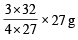= 24 g

Test: Single Correct MCQs: Some Basic Concepts of Chemistry | JEE Advanced - Question 2

### A compound was found to contain nitrogen and oxygen in the ratio 28 gm and 80 gm respectively. The formula of compound is (1978)

Detailed Solution for Test: Single Correct MCQs: Some Basic Concepts of Chemistry | JEE Advanced - Question 2

No. of nitrogen atoms =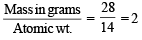No. of oxygen atoms =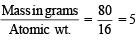∴ Formula of compound is N2O5.

Test: Single Correct MCQs: Some Basic Concepts of Chemistry | JEE Advanced - Question 3

### The largest number of molecules is in (1979)

Detailed Solution for Test: Single Correct MCQs: Some Basic Concepts of Chemistry | JEE Advanced - Question 3

(a) 18 g of H2O = 6.02 × 1023 molecules of H2O
∴ 36 g of H2O = 2 × 6.02 × 1023 molecules of H2O
= 12.04 × 1023 molecules of H2O
(b) 28 g of CO = 6.02 × 1023 molecules of CO
(c) 46 g of C2H5OH = 6.02 × 1023 molecules of C2H5OH
(d) 108 g of N2O5 = 6.02 × 1023 molecules of N2O5
∴ 54 g of N2O5 =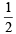× 6.02 × 1023 molecules of N2O5
= 3.01 × 1023 molecules of N2O5
∴ 36 g of water has highest number of molecules.

Test: Single Correct MCQs: Some Basic Concepts of Chemistry | JEE Advanced - Question 4

The total number of electrons in one molecule of carbon dioxide is

Detailed Solution for Test: Single Correct MCQs: Some Basic Concepts of Chemistry | JEE Advanced - Question 4

No. of e in C = 6 and in O = 8
∴ Total no. of e in CO2 = 6 + 8 × 2 = 22

Test: Single Correct MCQs: Some Basic Concepts of Chemistry | JEE Advanced - Question 5

A gaseous mixture contains oxygen and nitrogen in the ratio of 1 : 4 by weight. Therefore the ratio of their number of molecules is (1979)

Detailed Solution for Test: Single Correct MCQs: Some Basic Concepts of Chemistry | JEE Advanced - Question 5

Let mass of oxygen = 1g,
Then mass of nitrogen = 4g Mol. wt. of N2 = 28g, Mol. wt. of O2 = 32g
28 g of N2 has = 6.02 × 1023 molecules of nitrogen
4 g of N2 has =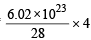molecules of nitrogen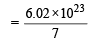molecules of nitrogen

32 g of O2 has = 6.02 × 1023 molecules of oxygen

∴ 1g of O2 has =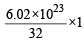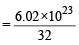moleculesof oxygen

Thus, ratio of molecules of oxygen : nitrogen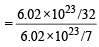= 7 : 32

Test: Single Correct MCQs: Some Basic Concepts of Chemistry | JEE Advanced - Question 6

2.76 g of silver carbonate on being strongly heated yields a residue weighing (1979)

Detailed Solution for Test: Single Correct MCQs: Some Basic Concepts of Chemistry | JEE Advanced - Question 6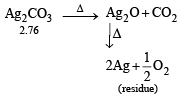NOTE : Ag2O is thermally unstable and decompose on heating liberating oxygen]
Mol. wt. of Ag2CO3 = 108 × 2 + 12 + 16 × 3 = 276 g
∴ 276 g of Ag2CO3 on heating gives residue      = 2 × 108 = 216 g of Ag
∴ 2.76 g of Ag2CO3 on heating gives =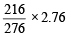= 2.16g of Ag

Test: Single Correct MCQs: Some Basic Concepts of Chemistry | JEE Advanced - Question 7

M is molecular weight of KMnO4. The equivalent weight of KMnO4 when it is converted into K2MnO4 is (1980)

Detailed Solution for Test: Single Correct MCQs: Some Basic Concepts of Chemistry | JEE Advanced - Question 7

The change involved is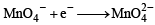i.e. it involves only one electron

Eq.wt =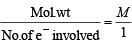= M [∵ Mol. wt. = M]

Test: Single Correct MCQs: Some Basic Concepts of Chemistry | JEE Advanced - Question 8

If 0.50 mole of BaCl2 is mixed with 0.20 mol of Na3PO4, the maximum number of moles of Ba3(PO4)2 that can be formed is (1981 - 1 Mark)

Detailed Solution for Test: Single Correct MCQs: Some Basic Concepts of Chemistry | JEE Advanced - Question 8

TIPS/Formulae : (i) Write balanced chemical equation for chemical change.
(ii) Find limiting reagent.
(iii) Amount of product formed will be determined by amount of limiting reagent.
The balanced equation is :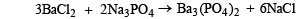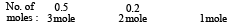Limiting reagent is Na3PO4 (0.2 mol), BaCl2 is in excess.
From the above equation : 2.0 moles of Na3PO4 yields Ba3(PO4)2 = 1 mole
∴ 0.2 moles of Na3PO4 will yield  Ba3(PO4)2 =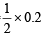= 0.1 mol.

Test: Single Correct MCQs: Some Basic Concepts of Chemistry | JEE Advanced - Question 9

One mole of N2H4 loses ten moles of electrons to form a new compound Y. Assuming that all the nitrogen appears in the new compound, what is the oxidation state of nitrogen in Y? (There is no change in the oxidation state of hydrogen). (1981 - 1 Mark)

Detailed Solution for Test: Single Correct MCQs: Some Basic Concepts of Chemistry | JEE Advanced - Question 9

TIPS/Formulae : (i) Find oxidation state of N in N2H4.
(ii) Find change in oxidation number with the help of number of electrons given out during formation of compound Y.
N2H4 → Y + 10 e, Calculation of O.S. of N in
N2H4 : 2x + 4 = 0  ⇒  x = –2 The two nitrogen atoms will balance the charge of 10 e.
Hence oxidation state of N will increase by +5, i.e. from –2 to +3.

Test: Single Correct MCQs: Some Basic Concepts of Chemistry | JEE Advanced - Question 10

The oxidation number of carbon in CH2O is (1982 - 1 Mark)

Detailed Solution for Test: Single Correct MCQs: Some Basic Concepts of Chemistry | JEE Advanced - Question 10

NOTE : The sum of oxidation states of all atoms in compound is zero.
Calculation of O.S. of  C in CH2O. x + 2 + (–2) = 0  ⇒ x = 0

Test: Single Correct MCQs: Some Basic Concepts of Chemistry | JEE Advanced - Question 11

A molal solution is one that contains one mole of a solute in: (1986 - 1 Mark)

Detailed Solution for Test: Single Correct MCQs: Some Basic Concepts of Chemistry | JEE Advanced - Question 11

TIPS/Formulae : Molality =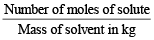A molal solution is one which contains one mole of solute per 1000 g of solvent.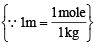Test: Single Correct MCQs: Some Basic Concepts of Chemistry | JEE Advanced - Question 12

The brown ring complex compound is for mulated as [Fe(H2O)5(NO)]SO4. The oxidation state of iron is :

Detailed Solution for Test: Single Correct MCQs: Some Basic Concepts of Chemistry | JEE Advanced - Question 12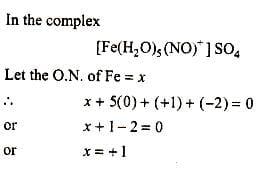Test: Single Correct MCQs: Some Basic Concepts of Chemistry | JEE Advanced - Question 13

The equivalent weight of MnSO4 is half of its molecular weight when it is converted to : (1988 - 1 Mark)

Detailed Solution for Test: Single Correct MCQs: Some Basic Concepts of Chemistry | JEE Advanced - Question 13

For equivalent weight of MnSO4 to be half of its molecular weight, change in oxidation state must be equal to 2. It is possible only when oxidation state of Mn in product is + 4. Since oxidation state of Mn in MnSO4 is + 2. So, MnO2 is correct answer.
In MnO2, O.S. of Mn = +4
∴ Change in O.S. of Mn = +4 – (+2) = +2

Test: Single Correct MCQs: Some Basic Concepts of Chemistry | JEE Advanced - Question 14

In which mode of expression, the concentration of a solution remains independent of temperature? (1988 - 1 Mark)

Detailed Solution for Test: Single Correct MCQs: Some Basic Concepts of Chemistry | JEE Advanced - Question 14

TIPS/Formulae : (i) Volume of substance changes with temperature and mass is not effected by change in temperature.
(ii) Find expression which does not have volume term in it.
(a) Molarity – Moles of solute/volume of solution in L.
(b) Normality – gm equivalents of solute/volume of solution in L.
(c) Formality – gm formula wt./volume of solution in L.
(d) Molality – Moles of solute/mass of solvent in kg
∵ Molality does not involve volume term.
∴ It is independent of temperature.

Test: Single Correct MCQs: Some Basic Concepts of Chemistry | JEE Advanced - Question 15

The oxidation number of phosphorus in Ba(H2PO2)2 is : (1990 - 1 Mark)

Detailed Solution for Test: Single Correct MCQs: Some Basic Concepts of Chemistry | JEE Advanced - Question 15

2 + 2 (2 + x – 4) = 0
[∵ Ba(H2PO2)2 is neutral molecule] or 2x – 2 = 0 ⇒ x = + 1

Test: Single Correct MCQs: Some Basic Concepts of Chemistry | JEE Advanced - Question 16

The oxidation states of the most electronegative element in the products of the reaction, BaO2 with dil. H2SO4 is (1991 - 1 Mark)

Test: Single Correct MCQs: Some Basic Concepts of Chemistry | JEE Advanced - Question 17

For the redox reaction :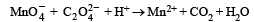the correct coefficients of the reactants for the balanced reaction are (1992 - 1 Mark)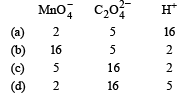Detailed Solution for Test: Single Correct MCQs: Some Basic Concepts of Chemistry | JEE Advanced - Question 17

TIPS/Formulae : Balance the reaction by ion electron method.
Oxidation reaction : C2 O 4-2 → 2CO2 + 2e] × 5
Reduction reaction : -
MnO-4 + 8H+ + 5e → Mn2+ + 4H2O] × 2
Net reaction : 2 MnO -4 + 16H+ + 5 C2O42- → 2Mn2+ + 10CO2 + 8H2O

Test: Single Correct MCQs: Some Basic Concepts of Chemistry | JEE Advanced - Question 18

The normality of 0.3 M phosphorous acid (H3PO3) is, (1999 - 2 Marks)

Detailed Solution for Test: Single Correct MCQs: Some Basic Concepts of Chemistry | JEE Advanced - Question 18

TIPS/Formulae :
(i) H3PO3 is dibasic acid as it contains two –OH groups.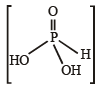(ii) Normality = Molarity × basicity of acid.
(iii) Basicity of H3PO3 = 2
∴   Normality = 0.3 × 2 = 0.6

Test: Single Correct MCQs: Some Basic Concepts of Chemistry | JEE Advanced - Question 19

The oxidation number of sulph ur in S8 , S2F2, H2 S respectively, are (1999 - 2 Marks)

Detailed Solution for Test: Single Correct MCQs: Some Basic Concepts of Chemistry | JEE Advanced - Question 19

TIPS/Formulae : (i) Oxidation state of element in its free state is zero.
(ii) Sum of oxidation states of all atoms in compound is zero.
O.N. of S in  S8 = 0;   O.N. of S in  S2F2 = + 1;
O.N. of S in  H2S = –2;

Test: Single Correct MCQs: Some Basic Concepts of Chemistry | JEE Advanced - Question 20

Amongst the following identify the species with an atom in +6 oxidation state (2000S)

Detailed Solution for Test: Single Correct MCQs: Some Basic Concepts of Chemistry | JEE Advanced - Question 20

TIPS/Formulae : (i) In an ion sum of oxidation states of all atoms is equal to charge on ion and in a compound sum of oxidation states of all atoms is always zero.
Oxidation state of Mn in MnO4 = + 7
Oxidation state of Cr in Cr(CN)63– = + 3
Oxidation state of Ni in NiF62– = + 4
Oxidation state of Cr in CrO2Cl2 = + 6

Test: Single Correct MCQs: Some Basic Concepts of Chemistry | JEE Advanced - Question 21

The reaction, 3ClO-(aq) → ClO 3- (aq) + 2Cl-(aq), is an example of (2001S)

Detailed Solution for Test: Single Correct MCQs: Some Basic Concepts of Chemistry | JEE Advanced - Question 21

TIPS/Formulae : (i) In a disproportionation reaction same elemen t undergoes oxidation as well as reduction during the reaction.
(ii) In decomposition reaction a molecule breaks down to more than one atoms or molecules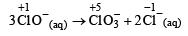It is disproportionation reaction because Cl is both oxidised (+ 1 to + 5) and reduced ( + 1 to – 1) during reaction.

Test: Single Correct MCQs: Some Basic Concepts of Chemistry | JEE Advanced - Question 22

An aqueous solution of 6.3 g oxalic acid dihydrate is made up to 250 ml. The volume of 0.1 N NaOH required to completely neutralize 10 ml of this solution is (2001S)

Detailed Solution for Test: Single Correct MCQs: Some Basic Concepts of Chemistry | JEE Advanced - Question 22

TIPS/Formulae : Equivalents of H2C2O4.2H2O = Equivalents of NaOH (At equivalence point)
Strength of H2C2O4 . 2H2O (in g/L) =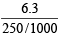= 25.2 g/L

Normality of H2C2O4 . 2H2O =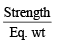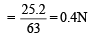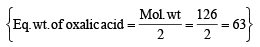Using normality equation : N1V1     =   N2V2
(H2C2O4.2H2O)     (NaOH)

0.4 × 10       =  0.1 × V2 or V2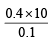= 40 ml.

Test: Single Correct MCQs: Some Basic Concepts of Chemistry | JEE Advanced - Question 23

In the standardization of Na2S2O3 using K2Cr 2O7 by iodometry, the equivalent weight  of K2Cr2O7 is (2001S)

Detailed Solution for Test: Single Correct MCQs: Some Basic Concepts of Chemistry | JEE Advanced - Question 23

TIPS/Formulae : (i) Find change in oxidation number of Cr atom.
(ii) Eq. wt. =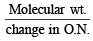In iodometry, K2Cr2O7 liberates I2 from iodides (NaI or KI).
Thus it is titrated with Na2S2O3 solution.
2Na2S2O3+ I2 → 2NaI + Na2S4O6 O.N. of Cr changes from + 6 (in K2Cr2O7) to +3. i.e. +3 change for each Cr atom
Cr2 O7-- + 14H+ + 6e- → 2Cr3++ 7H2O
Thus, one mole of K2Cr2O7 accepts 6 mole of electrons.
∴   Equivalent weight =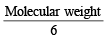Test: Single Correct MCQs: Some Basic Concepts of Chemistry | JEE Advanced - Question 24

How many moles of electron weigh one kilogram? (2002S)

Detailed Solution for Test: Single Correct MCQs: Some Basic Concepts of Chemistry | JEE Advanced - Question 24

TIPS/Formulae : (i) Mass of one electron = 9.108 × 10–31 kg
(ii) 1 mole of electron = 6.023 × 1023 electrons
Weight of 1 mole of electron = Mass of one electron × Avogadro Number = 9.108 × 10–31  × 6.023 × 1023 kg
∴ No. of moles of electrons in 1 kg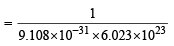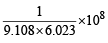Test: Single Correct MCQs: Some Basic Concepts of Chemistry | JEE Advanced - Question 25

Which has maximum number of atoms? (2003S)

Detailed Solution for Test: Single Correct MCQs: Some Basic Concepts of Chemistry | JEE Advanced - Question 25

TIPS/Formulae : Atomic weight in gms = 6.023 × 1023 atoms = 1 Mole atoms (i) Number of atoms in 24 g of C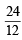× 6.023 × 1023 = 2 × 6.023 × 1023 atom

= 2 mole atoms

(ii) Number of atoms in 56 g of Fe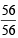× 6.023 × 1023 = 6.023 × 1023 atom   = 1 mole atoms

(iii) Number of atoms in 27 g of Al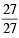× 6.023 × 1023 = 6.023 × 1023 atom   = 1 mole atoms

(iv) Number of atoms in 108 g of Ag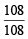× 6.023 × 1023 = 6.023 × 1023 atom   = 1 mole atoms

∴ 24 g of C has maximum number of atoms.

Test: Single Correct MCQs: Some Basic Concepts of Chemistry | JEE Advanced - Question 26

Mixture X = 0.02 mol of  [Co(NH3)5SO4]Br and 0.02 mol of [Co(NH3)5Br]SO4 was prepared in 2 litre of solution.  (2003S)
1 litre of mixture X + excess AgNO3 —→ Y.
1 litre of mixture X + excess BaCl2 —→ Z
No. of moles of Y and Z are

Detailed Solution for Test: Single Correct MCQs: Some Basic Concepts of Chemistry | JEE Advanced - Question 26

TIPS/Formulae : Write the reaction for chemical change during reaction and equate moles of products formed.
[Co(NH3)5SO4] Br has ionisable Br ions & [Co(NH3)5 Br] SO4 has ionisable SO4– – ion.

Given mixture X = 0.02 mol of [Co(NH3)5SO4] Br and 0.02 mol of [Co(NH3)5Br] SO4 Volume = 2 L
∴ Mixture X has 0.02 mol. of [Co(NH3)5SO4] Br and 0.02 mol of [Co(NH3)5Br] SO4 in 2 L of solution
∴ Conc. of [Co(NH3)5SO4] Br and [Co(NH3)5Br]SO4 = 0.01 mol/L for each of them.
(i) 1 L mixture of X + excess AgNO3 → Y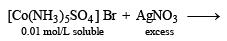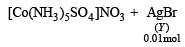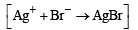∴ No. of moles of Y = 0.01

(ii) Also 1 L mixture of X + excess BaCl2 → Z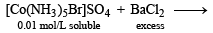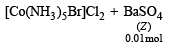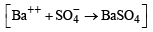∴ moles of Z = 0.01.

Test: Single Correct MCQs: Some Basic Concepts of Chemistry | JEE Advanced - Question 27

The pair of the compounds in which both the metals are in the highest possible oxidation state is (2004S)

Detailed Solution for Test: Single Correct MCQs: Some Basic Concepts of Chemistry | JEE Advanced - Question 27

TIPS/Formulae : The highest O.S. of an element is equal to the number of its valence electrons
(i) [Fe(CN)6]3–, O.N. of Fe = + 3,
[Co(CN)6]3–, O.N. of Co = + 3
(ii) CrO2Cl2, O.N. of Cr = +6, (Highest O.S. of Cr)
[MnO4]O.N of Mn = + 7 (Highest O.S. of Mn)
(iii) TiO3, O.N. of Ti = + 6, MnO2 O.N. of Mn = + 4
(iv) [Co(CN)6]3–, O.N. of Co = + 3, MnO3, O.N. of Mn = + 6

Test: Single Correct MCQs: Some Basic Concepts of Chemistry | JEE Advanced - Question 28

Consider a titration of potassium dichromate solution with acidified Mohr's salt solution using diphenylamine as indicator. The number of moles of Mohr's salt required per mole of dichromate is (2007)

Detailed Solution for Test: Single Correct MCQs: Some Basic Concepts of Chemistry | JEE Advanced - Question 28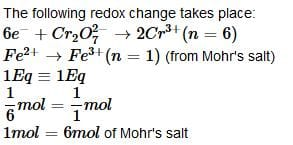## Chemistry for JEE

322 videos|586 docs|368 tests
 Use Code STAYHOME200 and get INR 200 additional OFF Use Coupon Code
Information about Test: Single Correct MCQs: Some Basic Concepts of Chemistry | JEE Advanced Page
In this test you can find the Exam questions for Test: Single Correct MCQs: Some Basic Concepts of Chemistry | JEE Advanced solved & explained in the simplest way possible. Besides giving Questions and answers for Test: Single Correct MCQs: Some Basic Concepts of Chemistry | JEE Advanced, EduRev gives you an ample number of Online tests for practice

## Chemistry for JEE

322 videos|586 docs|368 tests